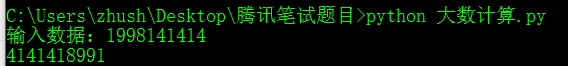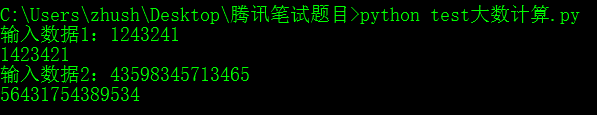# 腾讯后台开发面试题最后一道面试题题目以及答案：Python链表+大数计算（大数相加，完整代码在文章最后。）

2020年毕业生腾讯校园招聘提前批——后台开发——面试经验——分享给大家交流经验。

Pyhon3的链表的实现（包含遍历，读取，删除，头插，尾插）

# 基本思路：

MyList1 = SingleLinkList()
num = input("输入数据：")
for i in num:
MyList1.travel()# 正题开始：

MyList1 = SingleLinkList()
num = input("输入数据1：")
for i in num:
MyList1.travel()
num = input("输入数据2：")
for i in num:
MyList2.travel()# 上面的省略
"""一次读一个"""
while cur != None:
# print(cur.elem, end=' ')
yield cur.elem
cur = cur.next
yield None
# 下面的省略

MyList1_num = MyList1.read_next()
num1 = next(MyList1_num)
num2 = next(MyList2_num)

C1 = 0  # 代表进位
sum = 0 # 代表两个数字的和

ResList = SingleLinkList()

while num1 and num2:
sum = num1 + num2 + C1  # 求和
C1 = sum // 10
sum = sum % 10
num1 = next(MyList1_num)
num2 = next(MyList2_num)

if num1:
while num1:
C1 = (num1+C1) // 10
num1 = (num1+C1)%10
num1 = next(MyList1_num)
if num2:
while num2:
C1 = (num2+C1) // 10
num2 = (num2+C1)%10
num2 = next(MyList2_num)

if C1:
ResList.travel() # 遍历结果链表

# -*- coding:utf-8 -*-
class SingleNode(object):
"""单链表的节点"""
def __init__(self, elem):
self.elem = elem  # 存放数据元素
self.next = None  # 指向下一个数据元素

"""单链表的类"""
def __init__(self, node=None):
self.__length = 0  # 存放长度，因为这个程序里用不到，所以不需要也可
def is_empty(self):  # 可以删除，这个程序用不到
"""链表是否为空"""
def length(self):  # 可以删除，这个程序用不到
"""链表长度"""
return self.__length
def travel(self):  # 这个程序用到了这个
"""遍历整个链表"""
while cur != None:
print(cur.elem, end='')
cur = cur.next
print()
"""一次读一个"""
while cur != None:
# print(cur.elem, end=' ')
yield cur.elem
cur = cur.next
yield None
"""在头部添加元素, 头插法"""
node = SingleNode(item)
self.__length += 1

def append(self, item):  # 可以删除，这个程序用不到
"""在尾部添加元素,尾插法"""
node = SingleNode(item)
if self.is_empty():
else:
while cur.next != None:
cur = cur.next
cur.next = node
self.__length += 1

num = input("输入数据1：")
for i in num:
MyList1.travel()
num = input("输入数据2：")
for i in num:
MyList2.travel()

num1 = next(MyList1_num)
num2 = next(MyList2_num)
C1 = 0  # 代表进位
sum = 0 # 代表两个数字的和
while num1 and num2:
sum = num1 + num2 + C1  # 求和
C1 = sum // 10
sum = sum % 10
num1 = next(MyList1_num)
num2 = next(MyList2_num)
if num1:
while num1:
C1 = (num1+C1) // 10
num1 = (num1+C1)%10
num1 = next(MyList1_num)
if num2:
while num2:
C1 = (num2+C1) // 10
num2 = (num2+C1)%10
num2 = next(MyList2_num)
if C1:
ResList.travel() # 遍历结果链表


# 写在最后：

08-041万+
11-27216008-151249
11-275894
09-25302
08-102252
05-3150
07-318188
09-241514
04-13334